Latest Banking jobs   »

# Quantitative Aptitude Quiz For LIC AAO Mains 2023- 13th March

Q1. Arun daily works for X hours and earns Rs Y per hour. For each hour he works in excess of X hours, he is paid two times of his original rate. One day he works for Z hours and the amount he is paid on that day is Rs 1200 more than his daily amount. Find the possible value of (Z,X) if Y is either 100 or 150?
(i)12,8
(ii)10,4
(iii)9,5
(iv)13,7
(v)17,13
(a) Only (i) and (iii)
(b) Only (ii)and (iv)
(c) Only (ii) and (v)
(d) Only (i),(iii) and (v)
(e) All of the above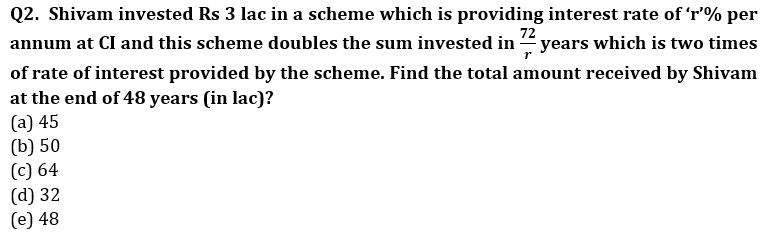Q3. A certain amount is invested in a scheme at CI of ‘p’% per annum compounded annually for ‘r’ years. Equal amount is invested in another scheme at CI of ‘q’% per annum compounded half-yearly for the same time period. If the interest earned from both schemes at the end of r years is same then which of the following can be the value of ‘p’ and ‘q’ if both are integers?
(i) 20,24
(ii) 24,30
(iii) 44,40
(iv) 21,20
(v) 12,16
(a) Only (ii) and (iv)
(b) Only (i),(ii)and (iv)
(c) Only (ii) and (iii)
(d) Only (iii) and (iv)
(e) Only (i),(ii) and (v)

Q4. A man invested in two different schemes A & B and investment in scheme A is 25% more than that of scheme B. Scheme A offered SI at the rate of (R – 2.5)% for two year while scheme B offered SI at the rate of (R + 5)% for three years and ratio of interest received by man from scheme A to that of scheme B is 5 : 12. Find the interest received by man, if he will invest Rs 2250 at the rate of 2R% per annum on CI for two years?
(a) 920 Rs
(b) 990 Rs
(c) 960 Rs
(d) 900 Rs
(e) 850 Rs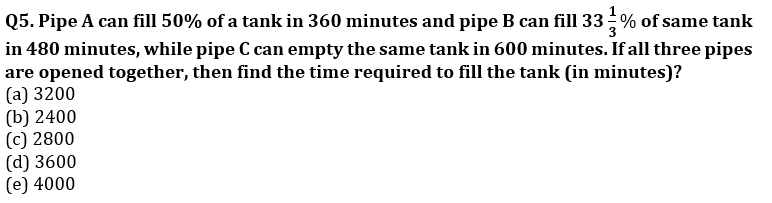Q6. A tank has four inlet pipes. Through the first three inlets pipes opened together, tank can be filled in 12 min and through the last three inlets, tank can be filled in 15 minutes and through the first and last inlets, tank can be filled in 24 minutes. Then find the time taken by last pipe to fill half of the tank?
(a) 80 min
(b) 60 min
(c) 40 min
(d) 20 min
(e) 30 min
Q7. Pipe A can fill a tank in 45 hr, pipe B is 50% more efficient than A and pipe C can fill the same tank in 7.5 hr less than B. A and B opened together after X hr both pipe closed and pipe C filled remaining tank in (X + 9) hr, if the ratio between tank filled by (A + B) together to tank filled by pipe C is 1 : 2. Find the value of X ?
(a) 2 hr
(b) 4 hr
(c) 12 hr
(d) 6 hr
(e) 8 hr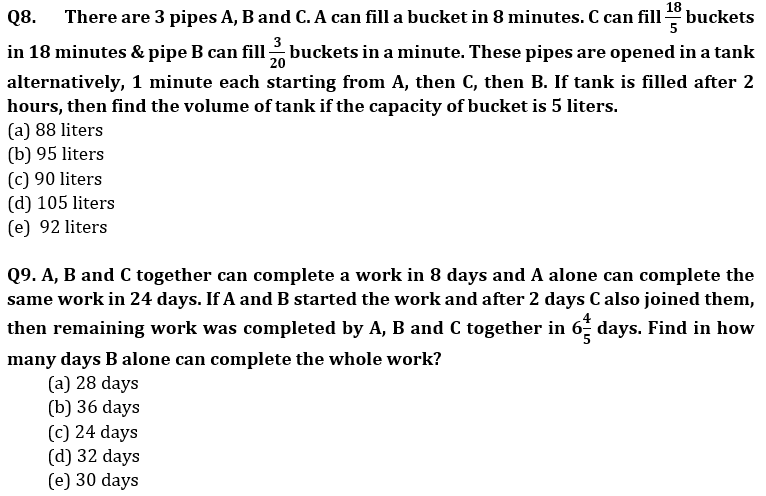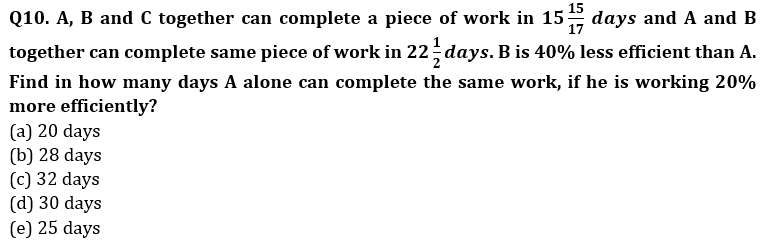Q11. Four persons started to do a work together. ‘A’ works only in starting two days after that B, C and D works alternately starting from B. Ratio of time taken by A, B, C and D if they work alone is 4 : 3 : 2 : 5. If the work is completed in 12 days then in how many days A and C can complete the work if they work together ?
(a) 6 days
(b) 12 days
(c) 10 days
(d) 8 days
(e) None of these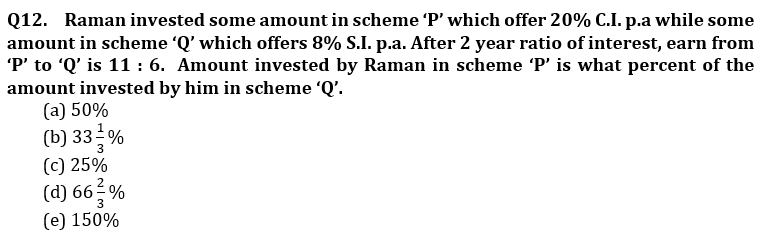Q13. A man invested an amount at the rate of 20% p.a. on compound interest for two years. After two years he added 75% of the initial amount to the amount obtained and invested this amount at same rate of interest. If man got a total of Rs. 3512 as interest after three years, find the amount received by man after three years, if he invested same amount on simple interest at rate of 15% p.a.?
(a) 5800 Rs.
(b) 5600 Rs.
(c) 5000 Rs.
(d) 5200 Rs.
(e) None of these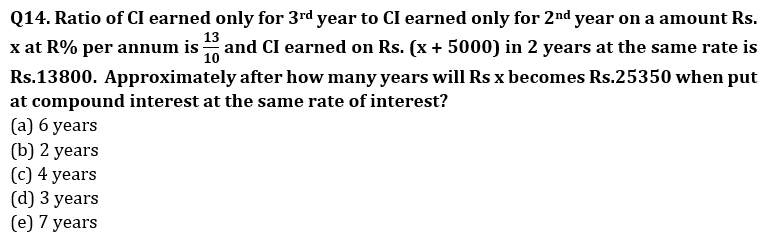Q15. Ritu invested her total saving in three different FD schemes A, B and C in the ratio of 5 : 4 : 6 on CI for two years at the rate of 10%, 15% and 20% respectively. If interest is calculated annually and interest from scheme B is Rs. 744 more than interest from scheme A then, find difference between interest received from scheme C and scheme B by Ritu?
(a) Rs. 4185
(b) Rs. 4175
(c) Rs. 3840
(d) Rs. 4580
(e) Rs. 3250

Solutions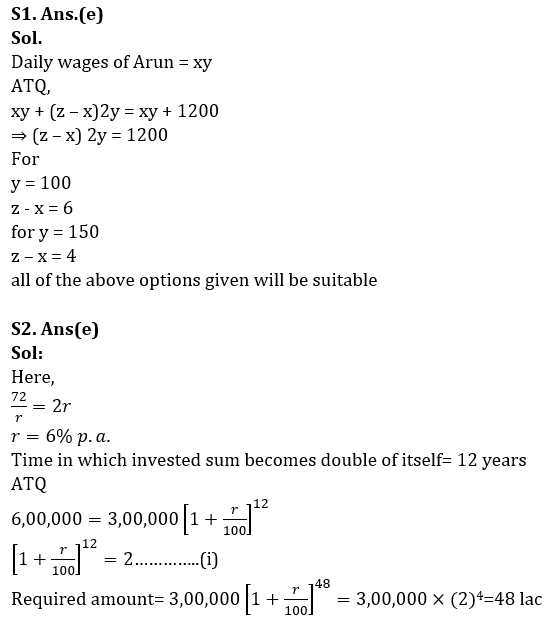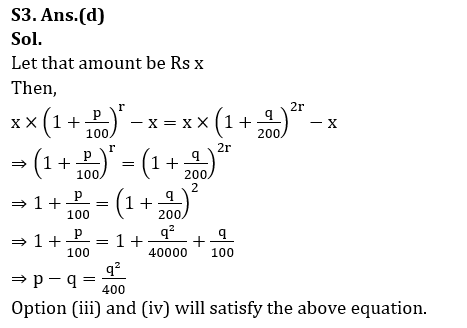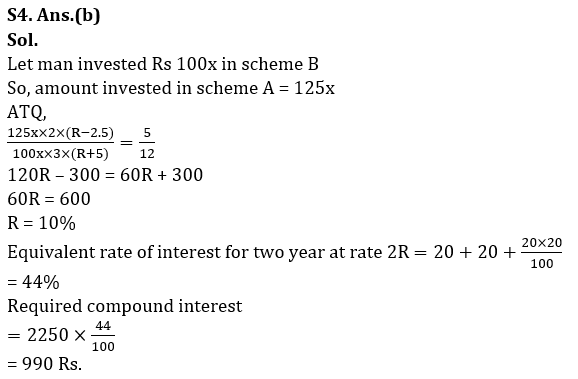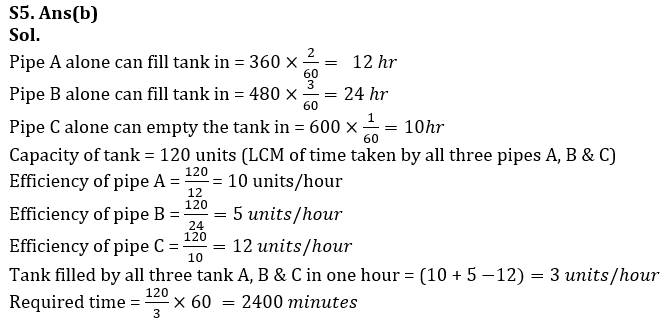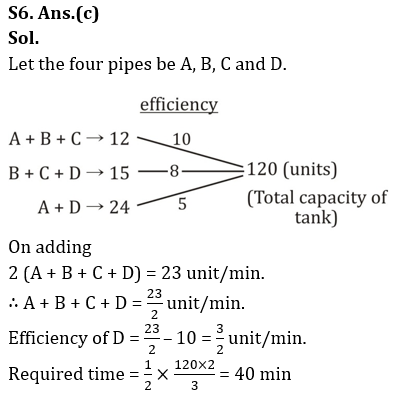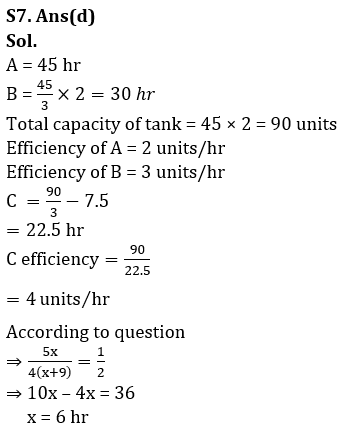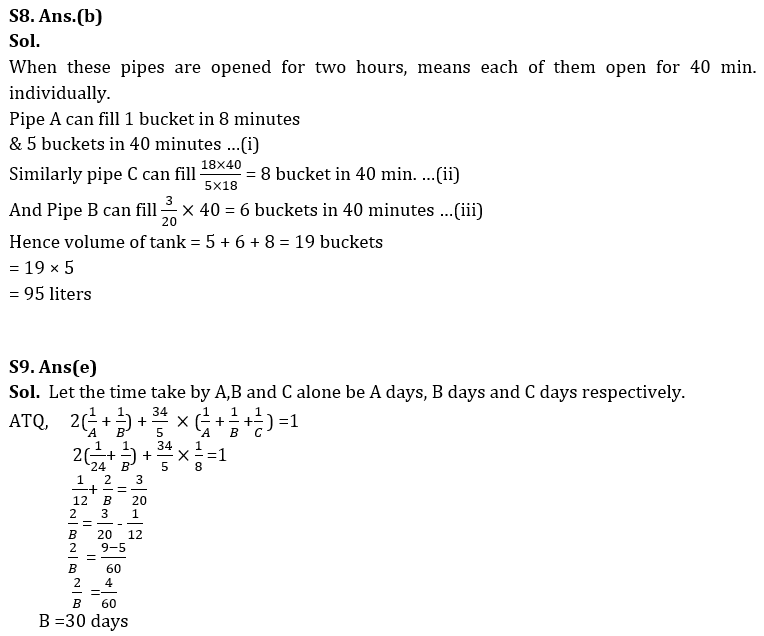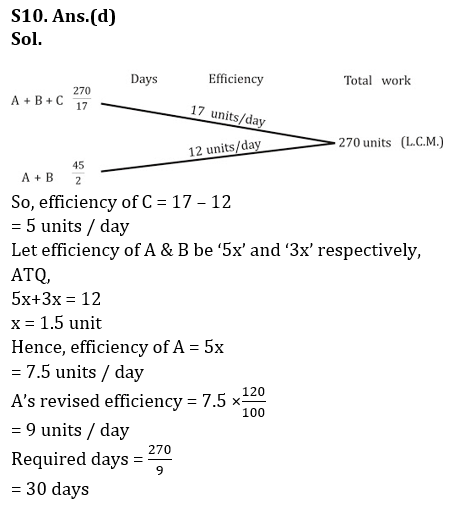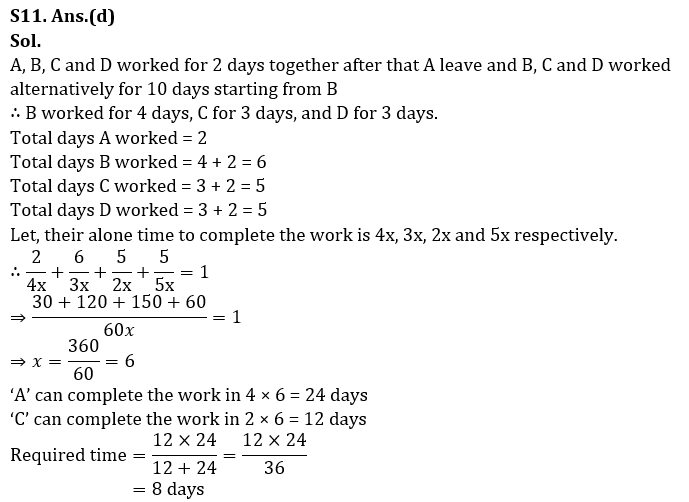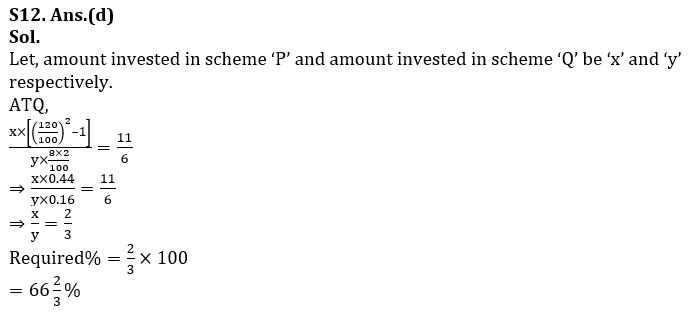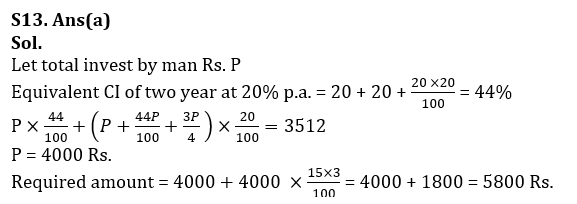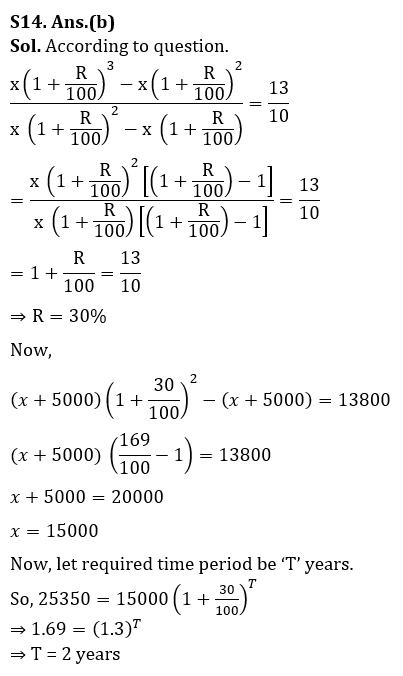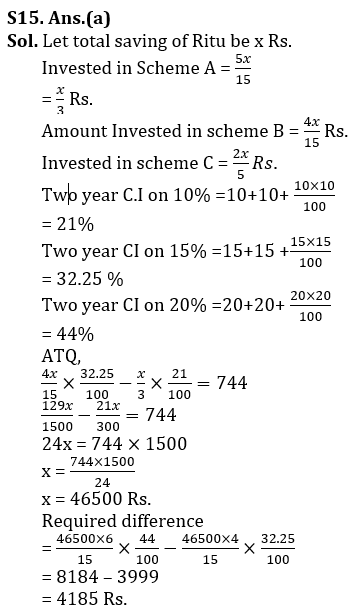## FAQs

### When will the LIC AAO Mains exam be held?

LIC AAO Mains exam will be held on 18 March

#### Congratulations!Union Budget 2023-24: Free PDF